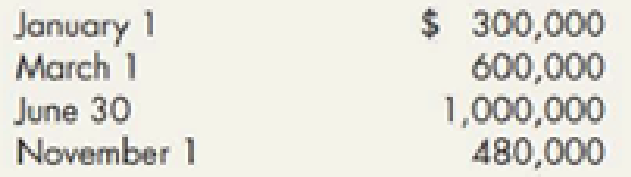Chapter 10, Problem 9RE### Intermediate Accounting: Reporting...

3rd Edition
James M. Wahlen + 2 others
ISBN: 9781337788281

#### Solutions

Chapter
Section### Intermediate Accounting: Reporting...

3rd Edition
James M. Wahlen + 2 others
ISBN: 9781337788281
Textbook Problem
17 views

# Dexter Construction Corporation is building a student condominium complex; it started construction on January 1, Year 1. Dexter borrowed \$2.5 million on January 1 specifically for the project by issuing a 10%, 5-vear, \$2.5 million note, which is payable on December 31 of Year 3. Dexter also had a 12%, 5-year, \$3 million note payable and a 10%, 10-year, \$1.8 million note payable outstanding all year. Calculate the weighted average interest rate on the non-construction-specific debt for Year 1.RE10-9 Refer to RE10-8. In Year 1, Dexter incurred costs as follows:Calculate Dexter’s weighted average accumulated expenditures.

To determine

Calculate the weighted average accumulated depreciation.

Explanation

Weighted average interest rate:

Weighted average interest rate is the total of the construction expenses weighted by the measure of time that interest cost is acquired on those expenditures during the period of construction.

Calculate weighted average expenditures:

 Expenditures × Portion of  year outstanding = Weighted average accumulated expenditures January 1 \$300,000 × 12/12 (January 1-December 31) = \$300,000 May...

### Still sussing out bartleby?

Check out a sample textbook solution.

See a sample solution

#### The Solution to Your Study Problems

Bartleby provides explanations to thousands of textbook problems written by our experts, many with advanced degrees!

Get Started